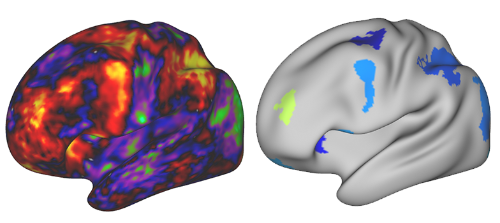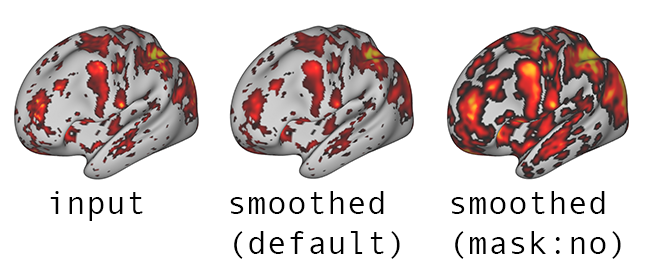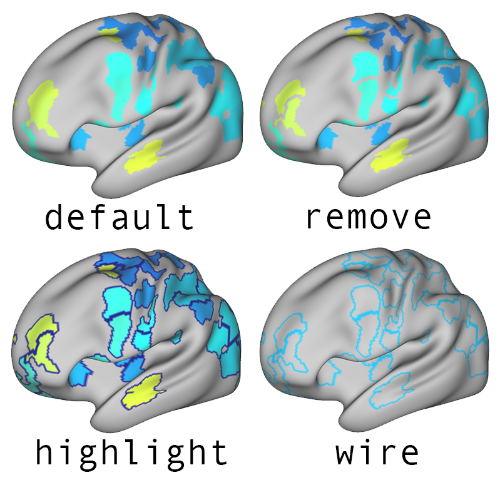# Identifying empirical ROI and extracting peak information#

## Overview#

Since the analysis of specific regions of interest (ROI) reduces the span of statistical analyses from the entire voxel space to specific regions of activation it is a common approach to fMRI activation data analysis. This approach has multiple benefits over analyzing the activation across the entire voxel space.

## Generating a ROI map from statistical maps#

ROI maps are generated with the QuNex command `general_find_peaks`, which is a part of `matlab/qx_mri/general`. The command takes a statistical map image as an input and outputs a map with generated ROIs. Prior to ROI computation, the input data can be additionally processed by performing the Gaussian smoothing and thresholding. ROIs are then computed with watershed segmentation algorithm. An example of a statistical map (input) and a generated ROI map (output) is shown in the image below.## general_find_peaks function description#

`general_find_peaks` is a wrapper to the `nimage.img_find_peaks()` method. The function generates a ROI map output file with the same format as the input image and a text report containing peak statistics.

```qunex general_find_peaks \
--fin="<path_to_the_input_file>" \
--fout="<path_to_the_output_file>" \
--mins="<minimum_size_and_area_of_generated_ROIs>" \
--maxs="<maximum_size_and_area_of_generated_ROIs>" \
--val="<thresholding_type>" \
--t="<thresholding_value>" \
--presmooth="<presmoothing_parameters>" \
--projection="<type_of_surface_projection_for_both_hemispheres>" \
--verbose="<whether_the_command_is_verbose>"
```

The breakdown of input parameters is as follows:

• `fin` (string) Path to the input file.

The file can be either:

• NIfTI (volumetric) file (`.nii.gz`), where data consists entirely of voxels, or

• CIFTI-2 (surface/volumetric) file (`.nii`), consisting of a vertex/voxel data.

CIFTI-2 images can either contain dense scalar data or dense data series. CIFT-2 files should therefore have extensions `dscalar.nii` or `dtimeseries.nii`. NIfTI files have `.nii.gz` extension, regardless of the temporal dimension.

Image is read with the `nimage()` function. For more information refer to help documentation for `nimage()`.

• `fout` (string) Path to the output file.

If the file with the same path already exists, it will be overwritten. The output file should have the same format as the input file (`fin`). For example, if the input file has a `dtseries.nii` extension, so must the output file.

Image is saved with the `nimage.img_saveimage()` method. For more information refer to `img_saveimage()` help documentation.

• `mins` (double or 1x2 double array) Minimum size and area of generated ROIs. Default: `mins = [0, 0]`

When processing NIfTI files, `mins` must be a scalar, defining the minimum size of volumetric ROIs in voxels, as shown below.

`mins = <minimum size of volumetric ROIs (vox)>`

When processing CIFTI-2 files, it must be a 1x2 array, where the first element defines the minimum size of volumetric ROIs (voxels) and the second element the minimum area of surface ROIs in square millimeters (mm^2), as shown below.

`mins = [<minimum size of volumetric ROIs (vox)>, <minimum area of surface ROIs (mm^2)>]`

• `maxs` (double or 1x2 double array) ​ Maximum size and area of generated ROIs. Default: `maxs = [Inf, Inf]`

When processing NIfTI files, `maxs` must be a scalar, defining the maximum size of volumetric ROIs in voxels, as shown below.

`maxs = <maximum size of volumetric ROIs (vox)>`

When processing CIFTI-2 files, it must be a 1x2 array, where the first element defines the maximum size of volumetric ROIs (voxels) and the second element the maximum area of surface ROIs in square millimeters (mm^2), as shown below.

`maxs = [<maximum size of volumetric ROIs (vox)>, <maximum area of surface ROIs (mm^2)>]`

• `val` (char) Thresholding type. Default: `val = 'b'`

`val` must be a character indicating whether the thresholding is applied to:

• positive values (`val = 'p'`), * negative values (`val = 'n'`) or

• the absolute value of the data (`val = 'b'`)

• `t` (double): Thresholding value. Default: `t = 0`

• `presmooth` (string) Presmoothing parameters. Default: `presmooth = []` (no smoothing).

`presmooth` must have the following format:

```presmooth = 'fwhm:[<VF> <SF>]|ftype:<TYPE>|ksize:<KS>
|wb_path:<PATH2WB>|hcpatlas:<PATH2HCP>'|timeSeries:<TS>|frames:<FLIST>'
```

where

• `fwhm` Full Width at Half Maximum information for volumetric and surface smoothing, passed either as a scalar or as a 2-element vector, where the first element (`<VF>`) defines the volumetric and the second element (`<SF>`) the surface fwhm value. For NIfTI image smoothing it should be a scalar. If a scalar is passed for CIFTI-2 image smoothing, it uses the same value for volumetric and surface smoothing.

• `ftype` Type of a smoothing kernel for volumetric data. Options are `'gaussian'` or `'box'`. Surface data smoothing is performed with the geodesic Gaussian method. The default kernel for volumetric data smoothing is `'Gaussian'`.

• `ksize` Smoothing kernel size for volumetric data, defined in voxels.

• `mask` Path to the mask image, which defines regions where smoothing is performed. If empty, it is by default set to be the same as the input file. If set to `mask:no`, no mask is used.

• `wb_path` Path to wb_command program (Connectome Workbench). This is required for surface data smoothing. If the path is stored in the `\$PATH` environment variable, then it is not required.

• `hcpatlas` Path to HCPATLAS folder containing projection (`surf.gii`) files. If `<HCPATLAS>` is stored as an environment variable `\$HCPATLAS`, then `hcpatlas` path is not required.

• `timeSeries` A boolean indicating whether a thresholded time series image should use each frame as a mask for the corresponding frame. By default the first frame is taken as a mask for all remaining frames.

• `frames` A list of frames passed as an array (e.g. [1 2 3]) to perform smoothing on. If left empty, it is by default set as `options.frames`.

`mask` parameter is particularly important in cases when a thresholded image is passed as an input to `general_find_peaks()`. If `mask` is unspecified, it by default uses the input (thresholded) image as a mask. If `mask` is explicitly specified as `mask:no`, then no mask is used and regions above the threshold are smeared into previously thresholded regions, as shown in the image below.Smoothing is performed with the `nimage.img_smooth()` method. For more information refer to `nimage.img_smooth()` documentation.

• `projection` (string) Type of surface projection for both hemispheres. Default: `projection = 'type:midthickness'`.

`projection` defines the surface projection for left and right cortex. This projection forms the basis for all surface data operations.

`projection` can be passed as:

• `'type:midthickness'`, `'type:inflated'`, etc. for default HCP surfaces or

• `'cortex_left:<CL_projection.surf.gii>|cortex_right:<CR_projection.surf.gii>'` to use specific `.surf.gii` files.

• `options` (string) Additional processing options. Default: `options = []` (only the first frame is processed and no boundary operations).

It must have the following format:

`options = 'frames:<FR>|boundary:<BTYPE>|parcels:<PTYPE>'`,

where

• `frames` - frames to be processed. Specific frames can be selected by passing an array of frames (e.g. [1 2 3]), use the string `all` to process all frames, if this parameter is empty ([]), only the first frame will be processed.

• `boundary` defines the values of each ROI boundary. It can be set to `remove` in order to set boundary values to 0, `highlight` to set boundary values to -100 or `wire`, where only ROI boundaries have non-zero values. An example of a file, processed 4 times with all 4 options for the boundary is shown on the image below.

• `parcels` defines whether to generate a text file reporting the composition of identified ROIs across CIFTI-2 volume structures (`parcels:volume`) or the composition across CIFTI-2 volume structures and across parcels defined in the input atlas image (`parcels:<path_to_atlas>`). The same report can be generated separately with the function `general_get_roi_parcels`. The output file is named (`<fin>_parcels.txt`). If this parameters is empty, no file is generated.

• `limitvol` defines whether to limit the growth of regions to subcortical structures as defined in CIFTI-2 format (applies to volume structures only). It can be set to `1` to limit the growth of regions. If set to `0` or left empty, the growth of regions is unaffected by CIFTI-2 subcortical region boundaries.• `verbose` (double) Defines whether the command is verbose or not. Default: `verbose = 0`.

`verbose` can be set as

• 0 - non-verbose,

• 1 - prints only information from the top-level command (`general_find_peaks()`) or * 2 - prints information from all subfunctions as well.

## Examples#

### NIfTI (volumetric) image#

To get a ROI image of both positive and negative peak regions with minimum z-values of (-)1, at least 50 contiguous voxels in size, but no larger than 250 voxels, after smoothing with a Gaussian kernel with `fwhm = 3` and `ksize = 6`, use:

```qunex general_find_peaks \
--fin="zscores.nii.gz" \
--fout="zscores_analyzed.nii.gz" \
--mins="50" \
--maxs="250" \
--val="b" \
--t=1 \
--presmooth="fwhm:3|ksize:6|ftype:gaussian" \
--verbose=2
```

To get a ROI image of both positive and negative peak regions with minimum z-values of (-)3, at least 72 contiguous voxels in size, but no larger than 300 voxels, after smoothing with a Gaussian kernel with `fwhm = 2` and `ksize = 7`, use:

```qunex general_find_peaks \
--fin="zscores.nii.gz" \
--fout="zscores_peaks_3_72_300.nii.gz" \
--mins="72" \
--maxs="300" \
--val="b" \
--t=3 \
--presmooth="fwhm:2|ksize:7" \
--verbose=1
```

### CIFTI-2 (surface/volumetric) image#

To get a ROI image of both positive and negative peak regions with minimum z-values of (-)3, at least 72 contiguous voxels in size or with minimum area of 80 mm^2, but no larger than 300 voxels or 350 mm^2, after smoothing with a kernel with `fwhm = 1` for volume and surfaces structures, where only frames 1, 6 and 7 will be processed, use:

```qunex general_find_peaks \
--fin="zscores.dtseries.nii" \
--fout="zscores_analyzed.dtseries.nii" \
--mins="[72 80]" \
--maxs="[300 350]" \
--val="b" \
--t=1 \
--presmooth="fwhm:3" \
--projection="type:inflated" \
--options="frames:[1 6 7]" \
--verbose=1
```

To get a ROI image of both positive and negative peak regions with minimum z-values of (-)3, at least 72 contiguous voxels in size or with minimum area of 80 mm^2, but no larger than 300 voxels or 350 mm^2, after smoothing with a kernel with `fwhm = 3` for volume and surfaces structures, where surface operations are based on specific surface files (`surf.gii`) for both hemispheres, use:

```qunex general_find_peaks \
--fin="zscores.dtseries.nii" \
--fout="zscores_peaks_3_72_300.dscalar.nii.gz" \
--mins="[72 80]" \
--maxs="[300 350]" \
--val="b" \
--t=3 \
--presmooth="fwhm:1" \
--projection="cortex_left:CL_projection.surf.gii|cortex_right:CR_projection.surf.gii" \
--verbose=1
```